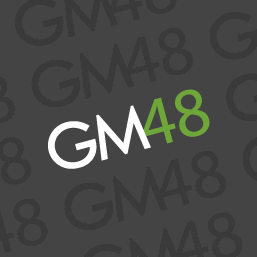← View other assets
asset

This shader is intended to approximate the effect of noise in low light photography with a high ISO.
######Lv. 21 SpritePainter
· 3 min read · 1670 views

1

``````attribute vec3 in_Position;                  // (x,y,z)
//attribute vec3 in_Normal;                  // (x,y,z)     unused in this shader.
attribute vec4 in_Colour;                    // (r,g,b,a)
attribute vec2 in_TextureCoord;              // (u,v)

varying vec2 v_vTexcoord;
varying vec4 v_vColour;

varying vec2 v_vPosition; //pass position data

void main()
{
vec4 object_space_pos = vec4( in_Position.x, in_Position.y, in_Position.z, 1.0);
gl_Position = gm_Matrices[MATRIX_WORLD_VIEW_PROJECTION] * object_space_pos;

v_vColour = in_Colour;
v_vTexcoord = in_TextureCoord;

v_vPosition = in_Position.xy; //get position
}``````

2

``````varying vec2 v_vTexcoord;
varying vec4 v_vColour;

varying vec2 v_vPosition;

uniform float seed; //seed passed in (increment in small values, ~0.0015 per step, for slower/more subtle effect)

float rand( vec2 p)
{
return fract( cos( dot( p, vec2(5.237,6.378)))* (8463.648 + seed));  //Xor's randomizing math with added seed modifier for animation
}

{
float x1 = rand(vec2(floor(p.x),floor(p.y)));
float x2 = rand(vec2(ceil(p.x),floor(p.y)));
float top = mix(x1,x2,smoothstep(0.0,1.0,fract(p.x)));

x1 = rand(vec2(floor(p.x),ceil(p.y)));
x2 = rand(vec2(ceil(p.x),ceil(p.y)));
float bottom = mix(x1,x2,smoothstep(0.0,1.0,fract(p.x)));

return mix(top,bottom,smoothstep(0.0,1.0,fract(p.y)));
}

void main()
{
gl_FragColor = texture2D( gm_BaseTexture, v_vTexcoord );  //incoming color data

float weighted = dot(gl_FragColor.rgb, vec3(0.299, 0.587, 0.114)); //weighted brightness value as found on
float n =
(noise(v_vPosition/1.0)*0.4 //smallest noise 40% of mix
+noise(v_vPosition/2.0)*0.3 //each line scales up and reduces % of mix to total 100% with 6 passes
+noise(v_vPosition/4.0)*0.1
+noise(v_vPosition/8.0)*0.1
+noise(v_vPosition/16.0)*0.1)
+ (sqrt(weighted)); //weighted brightness added to noise to blow out the brighter reas (reducing noise)

float dark_strength = 0.7; //increase to darken noise in bright areas
float light_strength = 0.5; //increase to lighten noise in dark areas

gl_FragColor *= (vec4(vec3(clamp(n,-0.2,1.2)),1.0)) * dark_strength; //controls the dark noise as it shows up in the brighter areas (multiply blending)
gl_FragColor += (vec4(vec3(clamp(1.0 - (n * 1.2),0.0,1.0) * 0.5),1.0)) * (1.0 - weighted) * light_strength; //additive pass to apply noise to dark areas
}``````

3
Implementation
To apply the shader to your entire scene, simply draw the application surface after all elements have been drawn that you want to have the effect. This can be done with the following call in the DrawGUI Event (shader asset name is "sh_noise"):

``````    shader_set(sh_noise);
draw_surface(application_surface,0,0); //redraw application surface with shader applied

The seed is set here so that it can be animated. Simply increment the variable each frame, smaller increments are better.

For a subtle effect I use `seed += 0.0015;` each step.

1
Credit
Credit for the noise shader code goes to Xor. For more information on how it works, check out their tutorials: